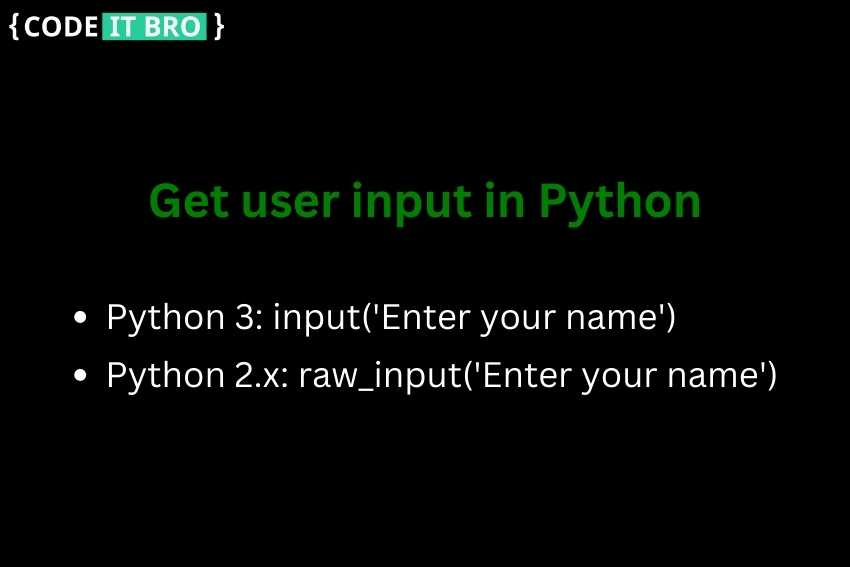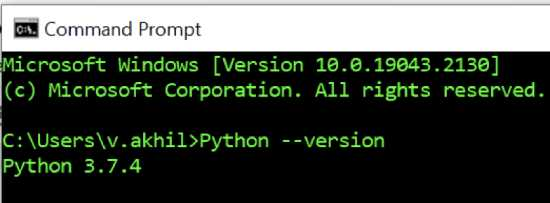# How to Get User Input in PythonWelcome to our tutorial on how to get user input in Python. In this blog, we will discuss the various ways to accept input from users in Python, including using built-in functions such as input() and raw_input().

By the end of this Python tutorial, you will have a solid understanding of how to get user input in Python and be able to apply it to your projects.

Let’s get started!

## How to Get User Input in Python

In Python 3, there is a built-in function called input() that allows the user to give input from the keyboard.

The entered input from the keyboard using the input() function is considered a type string by default.

After giving input from the keyboard, we need to press the Enter key. Then only the input() method considers/reads the data entered by the user.

Let’s now see the syntax of the input() function: `input(‘Enter Input’)`

The string specified in the input() function is printed on the console, and it’s optional.

Note: The program will halt indefinitely for the user input. There is no way to provide any timeout value.

Below is a simple example program to get input from the user.

```tutorial=input()
topic=input('Enter the Programming language-')
print(tutorial)
print(topic)```

Input:
CodeITBro

Enter the Programming language-Python

Output:

CodeITBro

Python

When a user gives input using the input() method, the default type of given input will be of type string.

Below is the sample Python program to find the type of variable.

```input1=input("Enter a number-")
print('Entered value -',input1,'is of type',type(input1))

input2=input("Enter a string-")
print('Entered value -',input2,'is of type',type(input2))```

Output:

Enter a number-143
Entered value – 143 is of type <class ‘str’>
Enter a string-I Love Bapatla
Entered value – I Love Bapatla is of type <class ‘str’>

Explanation: Even if the first input is a number, by default, it will consider it as a string, whatever we provide data as input from the input() function.

To consider the data taken from the input() function to a specific type like integer, we will use built-in type casting methods such as int(), float(), str(), etc.

```input1=int(input("Enter a Integer-"))
print('Entered value -',input1,'is of type',type(input1))

input2=float(input("Enter a floating point number-"))
print('Entered value -',input2,'is of type',type(input2))```

Output
Enter a Integer-72
Entered value – 72 is of type <class ‘int’>
Enter a floating point number-7.2
Entered value – 7.2 is of type <class ‘float’>

## How to get user input in Python 2 or earlier versions

In earlier versions, i.e., Python 2.x versions, the raw_input() function was used to take the input from the user.

Below is a simple example program that takes input from the user using the raw_input() method.

```input1=raw_input("Give input -")
print('The input taken from user is',input1)```

Output
Give input -Python 2
The input taken from user is Python 2

So use the input() function (or) raw_input() function based on the version of Python in the system.

To know the version of Python present in the system, follow the below steps:

1. Open the command prompt

2. Type this command: Python –versionBelow is an example program for reading the input from the user using the input() function.

```# total number of elements
length = int(input('Enter the count of elements required from user as input-'))

# list to store the content
list_of_elements = []

for i in range(0,length):
print('Enter the value',i+1)
element = input()
# append element into list
list_of_elements.append(element)

print(list_of_elements)```

Enter the count of elements required from the user as input-10
Enter the value 1
Bangalore
Enter the value 2
Mumbai
Enter the value 3
Delhi
Enter the value 4
Chennai
Enter the value 5
Lucknow
Enter the value 6
Punjab
Enter the value 7
Gujarat
Enter the value 8
Enter the value 9
Kolkata
Enter the value 10
Rajasthan
[‘Bangalore’, ‘Mumbai’, ‘Delhi’, ‘Chennai’, ‘Lucknow’, ‘Punjab’, ‘Gujarat’, ‘Hyderabad’, ‘Kolkata’, ‘Rajasthan’]

### Summary

Depending upon the Python version you are using, there are mainly two ways to get user input.

If you are using Python 3, you can use the input() function to store user input in your program. On the other hand, in earlier Python versions, you can use the raw_input() method.

Happy Coding!